## CALCULATING THE COEFFICIENT OF INBREEDING BY COANCESTRY

For complicated pedigrees (mainly found in animal breeding) the method of Coancestry offers an easier option than path analysis for calculating the Coefficient of Inbreeding. Coancestries are especially useful under systems of very close inbreeding where the number of inheritance pathways may be so great that identifying every one becomes too laborious. The Coefficient of Inbreeding of an individual (F) is equal to the Coancestry (f) between the two parents. In Figure 18:

FX = fPQ

Figure 18 Basic Formula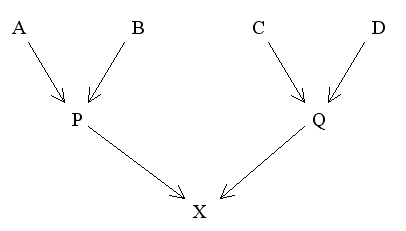fPQ = 1/4(fAC + fAD + fBC + fBD)

Supplementary Rules for Overlapping Generations

fPC = 1/2(fAC + fBC)

Also fPQ = 1/2(fPC + fPD)

Rules for Calculating Coancestries of Early Generations

Assume that all coancestries of the first generation are 0. The coancestries of the next generation will depend on the relationship between the prospective parents:

1. Selfing (Monoecious plants and hermaphrodites)
2. fAA = 1/2(1 + FA)

If FA is unknown or assumed to be 0 then fAA reduces to 1/2. The coancestry fAA is used again below in calculating coancestries between other closely-related parents.

3. Offspring and Parent
4. Since these are from different generations the supplementary rule is used:

fPA = 1/2(fAB + fAA)

If A and B are not related and A is not inbred, then fAB = 0 and f AA = 1/2 . The coancestry fPA then reduces to 1/4.

5. Full Sibs

The basic rule applies here, but the pedigree needs to be redrawn to find the formula.

Figure 19 Modified Diagram for Full SibsSince fAB = fBA then:

fPQ = 1/4(2fAB + fAA + fBB)

With no previous inbreeding or genetic relationship between A and B this reduces to fPQ = 1/4.

d) Half Sibs

Redrafting the pedigree as for full sibs:

Figure 20 Modified Diagram for Half Sibs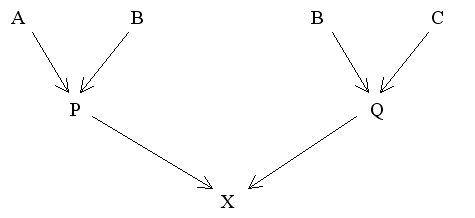Applying the basic rule to this diagram we have:

fPQ = 1/4(fAB + fAC + fBB + fBC)

With no previous inbreeding or genetic relationship between A and B or between B and C this reduces to fPQ = 1/8.

Worked Examples

With coancestries, unlike the previous path analyses, it is usual to start with the early generations and systematically work down through the pedigree until the parents of the chosen individual are reached. To be able to calculate the coancestries between actual parents it is also necessary to calculate the hypothetical coancestries between other members of each generation. Thus, a running tally is kept as you progress down through the generations. This avoids having to double back later to find missing values.

Two of the following four examples were used earlier to calculate coefficients of inbreeding by path analysis:

 Example Figure 1 21 First cousin marriages 2 22 Uncle - niece marriage 3 23 Double first cousin marriage 4 24 Close inbreeding (full sibs)

Example 1

Figure 21 First Cousin Marriages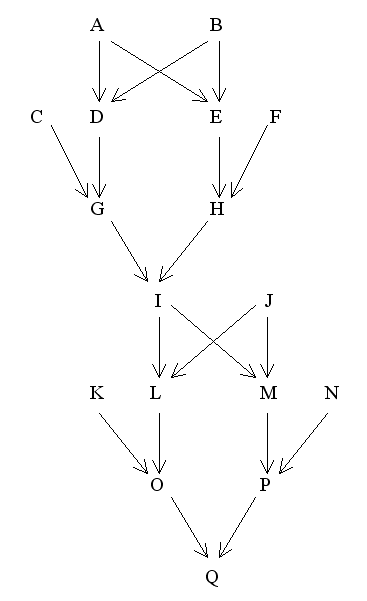Find FQ

The coancestries of the members of the early generations are as follows:

fAB = fCD = fCE = fCF = fDF = fEF = 0. Also FA = FB = FC = FD = FE = FF = 0.

Therefore, fDE (full sibs) = 1/4(2fAB + fAA + fBB) and since

fAA = 1/2(1 + FA) = 0.5 and fBB = 1/2(1 + FB) = 0.5

Then fDE = 1/4(0 + 0.5 + 0.5) = 0.25

fGH = FI = 1/4(fCE + fCF + fDE + fDF)

= 1/4(0 + 0 + 0.25 + 0)

= 0.0625

fIJ = fKL = fKM = fKN = fLN = fMN = 0

and FJ = 0

Therefore, fLM (full sibs) = 1/4(2fIJ + fII + fJJ)

fII = 1/2(1 + FI) = 0.53125 and fJJ = 1/2(1 + FJ) = 0.5

Therefore fLM = 1/4(0 + 0.53125 + 0.5) = 0.2578125

Finally, FQ = fOP = 1/4(fKM + fKN + fLM + fLN)

= 1/4(0 + 0 + 0.2578125 + 0)

= 0.064453125 = 6.45%

Example 2

Figure 22 Uncle - niece Marriage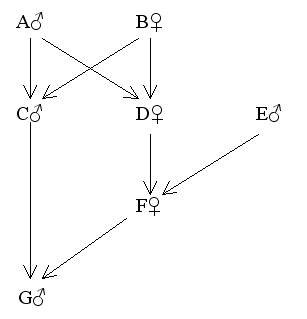Find FG

fAB = fCE = fDE = 0

Also FA = FB = FC = FD = FE = FF = 0

fCD (full sibs) = 1/4(2fAB + fAA + fBB)

Since fAA = 1/2(1 + FA) = 0.5 and fBB = 1/2(1 + FB) = 0.5

Then fCD = 1/4(0 + 0.5 + 0.5) = 0.25

Therefore FG = fCF (overlapping generations) = 1/2(fCD + fCE)

= 1/2(0.25 + 0)

= 0.125 = 12.5%

Example 3

Sometimes it is necessary, as shown earlier, to present a pedigree in alternative forms to facilitate the calculation of coancestries. The following are two different ways of depicting the same pedigree:

Figure 23 Double First Cousin Marriage

 Conventional DiagramModified Diagram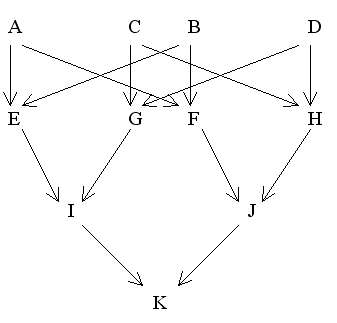Find FK

Using first diagram:

fAB = fAC = fAD = fBC = fBD = fCD = fEG = fEH = fFG = fFH = 0

Also FA = FB = FC = FD = FE = FF = FG = FH = 0

and fAA = 1/2(1 + FA) = fBB = 1/2(1 + FB) = fCC = 1/2(1 + FC) = fDD = 1/2(1 + FD) = 0.5

Then fEF (full sibs) = 1/4(2fAB + fAA + fBB)

= 1/4(0 + 0.5 + 0.5) = 0.25

and fGH (full sibs) = 1/4(2fCD + fCC + fDD)

= 1/4(0 + 0.5 + 0.5) = 0.25

Using second diagram

Therefore, FK = fIJ = 1/4(fEF + fEH + fGF + fGH)

= 1/4(0.25 + 0 + 0 + 0.25)

= 0.125 = 12.5%

Example 4

Figure 24 Close Inbreeding (full sibs)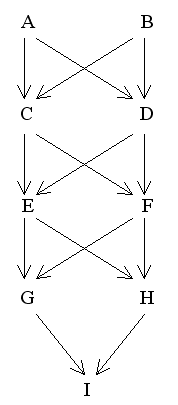Find FI

fAB = 0

FA = FB = FC = FD = 0

fCD (full sibs) = 1/4(2fAB + fAA + fBB)

fAA = 1/2(1 + FA) = 0.5 and fBB = 1/2(1 + FB) = 0.5

Therefore fCD = 1/4(0 + 0.5 + 0.5) = 0.25 = FE = FF

fEF (full sibs) = 1/4(2fCD + fCC + fDD)

fCC = 1/2(1 + FC) = 0.5 and fDD = 1/2(1 + FD) = 0.5

Therefore fEF = 1/4(0.5 + 0.5 + 0.5)

= 0.375 = FG = FH

fGH (full sibs) = 1/4(2fEF + fEE + fFF)

fEE = 1/2(1 + FE) = 0.625 and fFF = 1/2(1 + FF) = 0.625

Therefore, FI = fGH = 1/4(0.75 + 0.625 + 0.625)

= 0.5 = 50%

Alternative names for Coancestry are the Coefficient of Kinship, the Coefficient of Consanguinity and the Coefficient of Parentage. Lasley (1978) and Warwick and Legates (1979) employ a similar method to calculate F using covariance tables. The covariance between two prospective parents is equal to twice their coancestries and therefore twice the inbreeding coefficient of their progeny (F). For further information on coancestries see Falconer (1989).